Hydraulics in Civil Engineering

Hydraulics is an important field in Civil Engineering that has to do with the mechanical properties of liquids. Whether the project is a tunnel, road or series of pipes running through a building, it’s important to know how the water will travel and what conditions the building will be safe under. Read more about topics like fluid mechanics, fluid machinery, irrigation, coastal waters and more.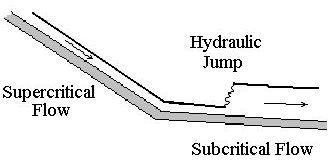Excel Formulas for Hydraulic Jump Calculations in a downloadable Excel Spreadsheet Templates

An Excel spreadsheet template that can be downloaded to make hydraulic jump calculations for open channel flow is available through this article. The Excel formulas in the template calculate Froude number and head loss, along with other hydraulic jump parameters.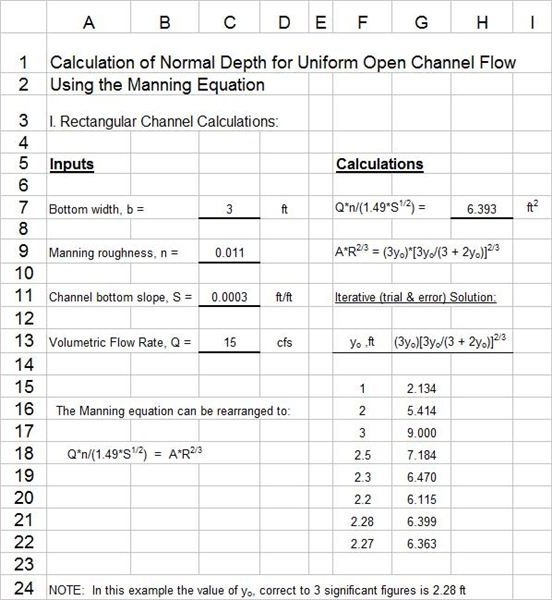Use of Excel Spreadsheets for Manning Equation/Normal Depth Calculation with Open Channel Flow

Find help calculating normal depth for uniform open channel flow with the downloadable templates available in this article. We use iterative Manning equation calculations to determine the normal depth of flow that will take place for a given flow rate, in a channel of known bottom slope, size, shape, and roughness.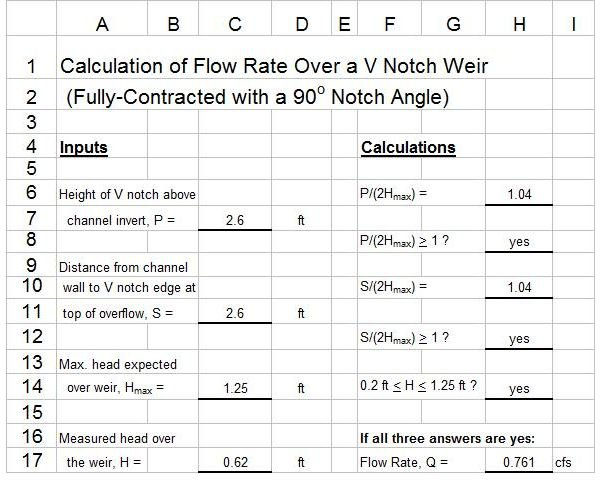Open Channel Flow Measurement/V Notch Weir Calculations with Excel Spreadsheet Templates

The Excel spreadsheet templates provided in this article will help you make open channel flow measurement calculations for water flow rate over a V notch weir. Formulas are given for the simple calculations of a 90 degree V notch weir and the more complicated calculations for a non 90 degree notch angle.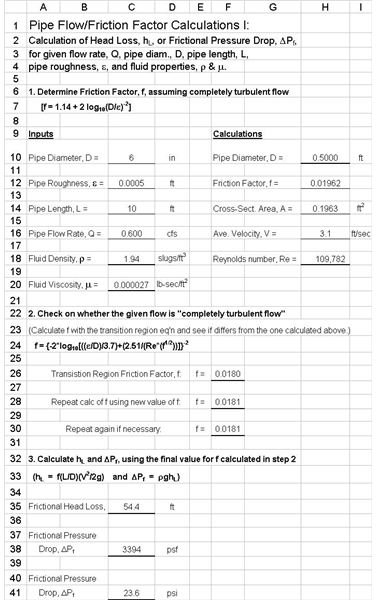The Use of Excel Spreadsheet Templates for Pipe Flow Calculations Such as Head Loss, Pressure Drop, or Pipe Diameter

Calculation of frictional head loss or pressure drop for pipe flow, using the Darcy Weisbach/ friction factor equation, can be done with downloadable Excel spreadsheet templates given in this article. A template is also given for calculation of pipe diameter needed for given flow rate and head loss.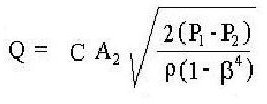Use Excel Formulas for Orifice, Venturi Meter and Ideal Gas Law Calculations

Excel templates can be downloaded to use Excel formulas for venturi meter, orifice flow meter and ideal gas law calculations. The Excel formulas calculate pipe flow rates for venturi and orifice flow meters, gas density with the ideal gas law, and orifice coefficient with the ISO 5167 equation.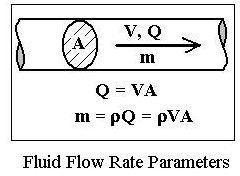Conversion of Linear Velocity to Volumetric Flow Rate or to Mass Flow Rate

When using the continuity equation for fluid mechanics calculations, a conversion of linear velocity to volumetric flow rate is sometimes needed for flow in pipes or for open channel flow. See continuity equation calculations, and examples of conversions among different measures of fluid flow rate.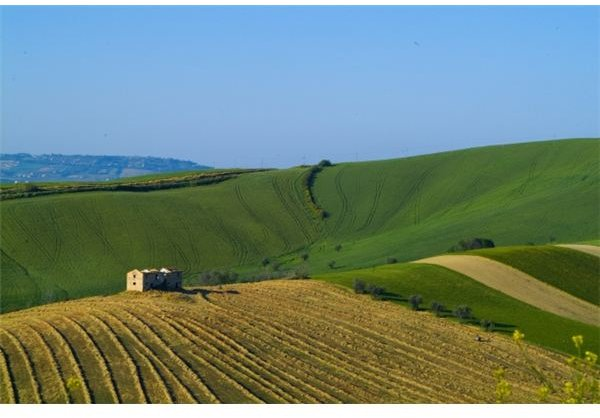How Agricultural Hydrogels Help To Conserve Water: Learn How They’re Made & Used

Agricultural hydrogels are synthetic polymers generally made from petroleum products. They absorb many times their weight in water, and can be distributed into dry regions in order to improve the soil’s ability to absorb water. Learn how they’re made and how they can be used.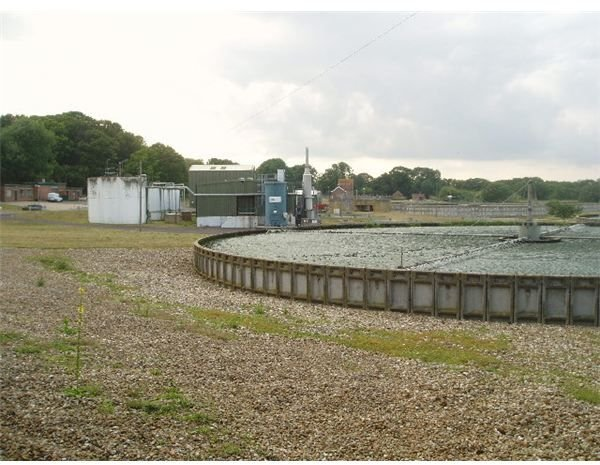Wastewater Ozone Treatment: an Environmentally Sound Option

Ozone is a form of oxygen that readily gives up its additional atom to react with bacteria, molds, and other pollutants to oxidize them and render them less harmful. Wastewater ozone treatment is thus one of the advanced wastewater treatments that is considered worldwide for its effectiveness.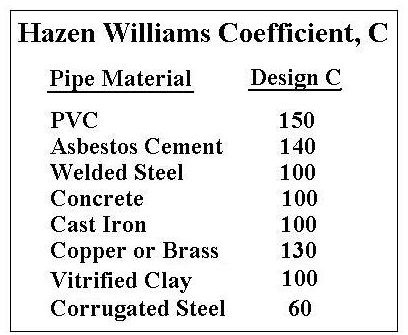Water Flow Rates for Pipe Sizes with Excel Formulas, Using the Hazen Williams Formula

Excel formulas to calculate water flow rates for pipe sizes (diameters and lengths) can be downloaded as Excel templates in this article. The Hazen Williams formula is used for water flow rate calculations. Either S.I. units or U.S. units can be used in the Excel spreadsheet templates.

Severn Tidal Barrage

There have been numerous government enquiries and subsequent reports over the last century into the construction of a tidal barrage across the River Severn Estuary linking England to Wales.
None have come to fruition due to economic and environmental concerns and, the sheer magnitude of the project

Open Channel Flow Calculations Include Determining Manning Roughness Coefficient n equation.

Determining Manning roughness coefficient, n, for use in the Manning equation, is a key step in open channel flow calculations for water flow in a natural channel. Determining the Manning roughness coefficient, n, for a natural channel is difficult due to the variability of channel surfaces.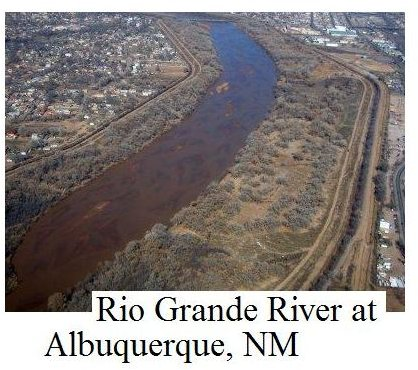Manning Equation Flow Calculator for Open Channel Flow Measurement

The Manning equation is widely used for open channel flow calculations. It can be used for water flow rate and flow velocity calculations in man made open channels, and for river discharge and river flow velocity in terms of the slope, size & shape and roughness characteristics of natural channels.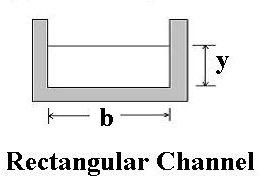Calculation of Open Channel Flow Hydraulic Radius: Calculate using Trapezoid Area

The hydraulic radius for different channel cross-sections, such as a trapezoid, triangle or rectangle shape, is used for Manning equation and open channel flow calculations. Trapezoid area and perimeter and triangle area and perimeter, for example are needed to calculate the hydraulic radius.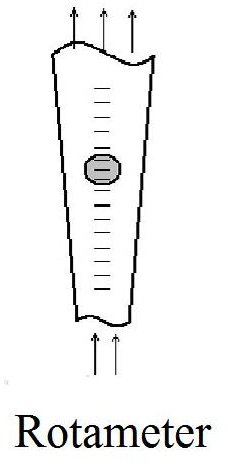Rotameter Flow Meters for Water Pipe Flow Rates Measurement

Values of pipe flow rate are needed for many purposes. Pipe flow measurement can be done with various types of flow meter, including the rotameter, which is covered in this article. The principle of operation and advantages and disadvantages of the rotameter for pipe flow measurement are discussed.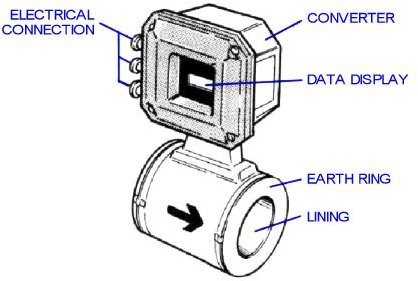Magnetic Flow Meter: Measurement & Calculations of Flow Rate

The magnetic flow meter is a convenient way to measure pipe flow rate. A magnetic flow meter has no internal parts, so it creates no pressure drop as part of its pipe flow measurement. A magnetic flow meter does have a high cost and power requirement compared to other types of pipe flow meter.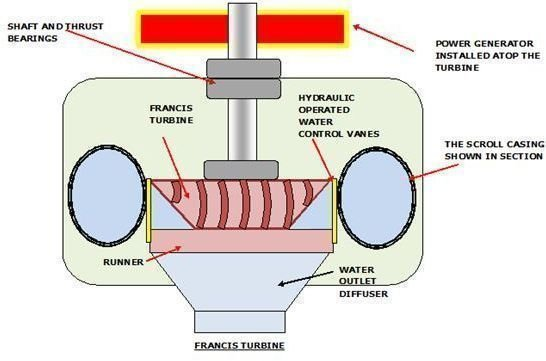Hydrokinetic Energy From Coaxial Water Reaction Turbines

A reaction water turbine is used where the water supplied from the dam is of low head combined with a high flow quantity. There are two types of reaction turbines used in hydrokinetics; propeller and radial turbines. Both types are enclosed in a casing similar in shape to that of a centrifugal pump.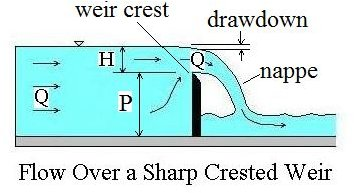Use a Rectangular Weir to Measure Water Flow Rate in an Open Channel

A rectangular weir is used to meter flow in an open channel. The head over the rectangular weir is measured and correlated with the water flow rate through the open channel (and over the weir). A rectangular weir equation gives water flow rate as a function of head over the rectangular weir.